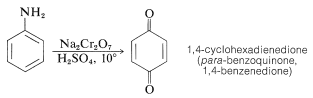# 23.11: Oxidation of Amines

$$\newcommand{\vecs}{\overset { \rightharpoonup} {\mathbf{#1}} }$$ $$\newcommand{\vecd}{\overset{-\!-\!\rightharpoonup}{\vphantom{a}\smash {#1}}}$$$$\newcommand{\id}{\mathrm{id}}$$ $$\newcommand{\Span}{\mathrm{span}}$$ $$\newcommand{\kernel}{\mathrm{null}\,}$$ $$\newcommand{\range}{\mathrm{range}\,}$$ $$\newcommand{\RealPart}{\mathrm{Re}}$$ $$\newcommand{\ImaginaryPart}{\mathrm{Im}}$$ $$\newcommand{\Argument}{\mathrm{Arg}}$$ $$\newcommand{\norm}{\| #1 \|}$$ $$\newcommand{\inner}{\langle #1, #2 \rangle}$$ $$\newcommand{\Span}{\mathrm{span}}$$ $$\newcommand{\id}{\mathrm{id}}$$ $$\newcommand{\Span}{\mathrm{span}}$$ $$\newcommand{\kernel}{\mathrm{null}\,}$$ $$\newcommand{\range}{\mathrm{range}\,}$$ $$\newcommand{\RealPart}{\mathrm{Re}}$$ $$\newcommand{\ImaginaryPart}{\mathrm{Im}}$$ $$\newcommand{\Argument}{\mathrm{Arg}}$$ $$\newcommand{\norm}{\| #1 \|}$$ $$\newcommand{\inner}{\langle #1, #2 \rangle}$$ $$\newcommand{\Span}{\mathrm{span}}$$$$\newcommand{\AA}{\unicode[.8,0]{x212B}}$$

## Oxidation States of Nitrogen in Organic Compounds

Nitrogen has a wide range of oxidation states in organic compounds. We can arrive at an arbitrary scale for the oxidation of nitrogen in much the same way as we did for carbon (Section 11-1). We simply define elementary nitrogen as the zero oxidation state, and every atom bonded to nitrogen contributes -1 to the oxidation state if it is more electropositive than nitrogen (e.g., $$\ce{H}$$, $$\ce{C}$$, $$\ce{Li}$$, $$\ce{B}$$, $$\ce{Mg}$$) and +1 if it is more electronegative (e.g., $$\ce{O}$$, $$\ce{F}$$, $$\ce{Cl}$$). Doubly bonded atoms are counted twice, and a formal positive charge associated with nitrogen counts as +1.

To illustrate, the oxidation states of several representative compounds are as follows:Several types of nitrogen compounds are listed in Table 23-5 to illustrate the range of oxidation states that are possible.

Table 23-5: Oxidation States of Nitrogen## Oxidation of Tertiary Amines. Amine Oxides

For the oxidation of a tertiary amine by reagents such as hydrogen peroxide, $$\ce{H_2O_2}$$, or peroxycarboxylic acids, $$\ce{RCOOOH}$$, which can supply an oxygen atom with six electrons, the expected product is an azane oxide (amine oxide). Thus $$\ce{N}$$,$$\ce{N}$$-diethylethanamine (triethylamine) can be oxidized to triethylazane oxide (triethylamine oxide):Amine oxides are interesting for two reasons. First, amine oxides decompose when strongly heated, and this reaction provides a useful preparation of alkenes. With triethylazane oxide (triethylamine oxide), ethene is formed:The second interesting point about amine oxides is that, unlike amines, they do not undergo rapid inversion at the nitrogen atom, and the oxides from amines with three different $$\ce{R}$$ groups are resolvable into optically active forms. This has been achieved for several amine oxides, including the one from $$\ce{N}$$-ethyl-$$\ce{N}$$-methyl-2-propanamine.

## Oxidation of Primary and Secondary Alkanamines

Addition of an oxygen atom from hydrogen peroxide or a peroxyacid to a primary or secondary amine might be expected to yield an amine oxide-type intermediate, which then could rearrange to an azanol (hydroxylamine):However, these oxidations usually take a more complicated course, because the azanols themselves are oxidized easily, and in the case of primary amines, oxidation occurs all the way to nitro compounds, in fair-to-good yields:## Oxidation of Aromatic Amines

We shall use benzenamine to illustrate some typical oxidation reactions of arenamines. The course of oxidation depends on the nature of the oxidizing agent and on the arenamine. With hydrogen peroxide or peroxycarboxylic acids, each of which functions to donate oxygen to nitrogen, oxidation to the azanol, the nitroso, or the nitro compound may occur, depending on the temperature, the pH, and the amount of oxidizing agent:Oxidizing agents that abstract a hydrogen atom or hydride ion lead to more complex reactions, which often result in highly colored products. One of the best black dyes for fabric (Aniline Black) is produced by impregnating cloth with phenylammonium chloride solution and then oxidizing, first with sodium chlorate $$\left( \ce{NaClO_3} \right)$$ and finally with sodium dichromate $$\left( \ce{Na_2Cr_2O_7} \right)$$. Aniline Black probably is not a single substance, and its exact structure(s) is not known; but its formation certainly involves addition reactions in which carbon-nitrogen bonds are made. A possible structure is shown in which there are seven aniline units:Oxidation of benzenamine with sodium dichromate in aqueous sulfuric acid solution produces 1,4-cyclohexadienedione (para-benzoquinone), which is the simplest member of an interesting class of conjugated cyclic diketones that will be discussed in more detail in Chapter 26: